## Social Question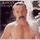# What do you think is the most mind-blowing concept in mathematics?

Asked by cockswain (15271) April 11th, 2011

I was just watching a series of calculus videos on KhanAcademy.org, and over about 1hr he shows how the infinite series representation of sin x and cos x are closely related to the infinite series representation of e^x. e is a pretty wild number for many reasons, not the least being the fact that it’s derivative is e^x. Anyways, when one compares the infinite sums, a pattern emerges, and eventually Khan shows that e^(ix) = cos x + i sin x, where i is the imaginary number. Finally, e^(i pi) = -1. This is a pretty wild fact about the universe, that these numbers from several disciplines of mathematics can be drawn together for such a simple, elegant conclusion.

This is a seven part series. Here is part 6, where things start getting pretty interesting.

So that currently gets my vote for most fascinating mathematical concept. What do you think is the coolest thing?

Observing members: 0Composing members: 0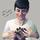Infinity in general is pretty grand. Sometimes, if you take a graph with an infinite area under the curve, revolve that curve around the x axis to get a three dimensional shape, and then calculate the volume of that shape, oddly enough you might get a finite volume. Dimensionality is WEIRD.

Also, just the thought that some infinities are bigger than others. For instance, there’s an infinite number of even numbers, but there’s a larger infinite number of integers.

Also a fan of Euler’s Identity though. I left my calculus lecture feeling all tingly the day we learned that one.

Mariah (25876)“Great Answer” (2) Flag as…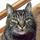I too have had my mind blown in dealing with the concept of the exponential function of y=e^x when I took a semiconductor electronics class. Where e is about 2.718281828 and the variable x is usually a complex number. I used this information as a tool but failed to truly understand it. Fun stuff.

gondwanalon (19906)“Great Answer” (1) Flag as…Yes. And just like 1 = .9 + .09 +.009 + .0009… , e = the sum of the series 1/n! from 0 to inf. Pretty wild to think like that. Nothing is really whole, just comprised of infinite sums.

cockswain (15271)“Great Answer” (3) Flag as…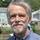There are tons of things coming out in math today that are mind blowing. But the all-time world record, IMHO, goes to Sir Isaac Newton. Somebody asked him why the planets followed elliptical orbits. With his theory of gravity already in his head, he went away for two months to think about an answer to the question. When he returned with the answer, he had not only described a very accurate model of the motion of orbital objects under the influence of mass attraction, he had invented calculus because he needed that to answer the question.

I suspect he may have been the most brilliant human who ever walked the face of this Earth.

ETpro (34552)“Great Answer” (3) Flag as…Yes, Newton is pretty underestimated by the layman. People just think “oh, he figured out a few physics equations,” but he did so much.

It’s amazing what people figured out without computers in past centuries. Every math book has footnotes about these guys who discovered important fundamental equations in the 1700s, or 1800s. They had nothing but passion to discover these things. Any other life would have been easier, but doubtlessly less satisfying.

There’s a modern physicist, Lisa Randall, who has an amazing mind. Cute too. Also Edward Witten gets my vote for one of the smartest ever.

cockswain (15271)“Great Answer” (1) Flag as…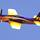The fact that Fermat’s Last Theorem took 358 years to prove.

And personally, I think it’s kind of awesome you’re watching calculus videos. You and I would have a lot to talk about over a beer.

Rarebear (25162)“Great Answer” (1) Flag as…I’ll most likely be in San Jose in August for a week or two.

cockswain (15271)“Great Answer” (0) Flag as…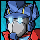Differential Equations.

weeveeship (4637)“Great Answer” (0) Flag as…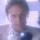The real numbers are pretty far out. I thought I had a firm grasp on them coming through the usual (applied) math sequence as an engineering student at a Big Ten university. Then latter in life I took some pure math courses and was thoroughly disabused of this notion.

The most mind blowing thing I was able to convince myself of totally in a mathematical sense was “constructing” a cover for the all the rationals on the unit interval that could be made as small as you wanted. Yet any finite interval of the real line has infinitely many rationals within it. Add to this the fact that the rationals are countable, while the extremely dispersed “dust” you get with Cantor’s middle third construction is uncountable (on the same order as the reals themselves).

hiphiphopflipflapflop (6100)“Great Answer” (1) Flag as…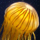The whole bally lot of it! I learnt most of my calculations by playing darts/snooker/golf. A fun way of keeping the grey matter busy.

ucme (50034)“Great Answer” (0) Flag as…cool. Let me know when and maybe Rocketguy and I can meet up with you. He lives in San Jose.

Rarebear (25162)“Great Answer” (0) Flag as…will do.

cockswain (15271)“Great Answer” (0) Flag as…@hiphiphopflipflapflop That tiny finite interval of the real having infinite rationals is so irrational, and the infinity of reals are just so unreal!

ETpro (34552)“Great Answer” (0) Flag as…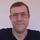@Mariah – There’s an infinite number of even numbers, but the infinite number of integers is not larger and neither is the infinite number of fractions (rational numbers). Cantor showed why this is so.

What is mind-blowing is that the innumerable number of real numbers is in fact larger than the infinite number of rational numbers. How can there be different levels of infinity? Amazing, right. Maybe that’s what you meant.

Here’s another mind-blowing concept: Ackermann numbers.

http://en.wikipedia.org/wiki/Ackermann_function#Ackermann_numbers

The n-th Ackermann number is defined to be n↑^n n, so

1↑1 = 1^1 = 1,
2↑↑2 = 2↑2 = 2^2 = 4

So how much bigger is 3↑↑↑3?

The result is mind boggling. Try writing it down using one Planck length for each digit. You gonna be looking for a lot of new universes.

mattbrowne (31643)“Great Answer” (0) Flag as…